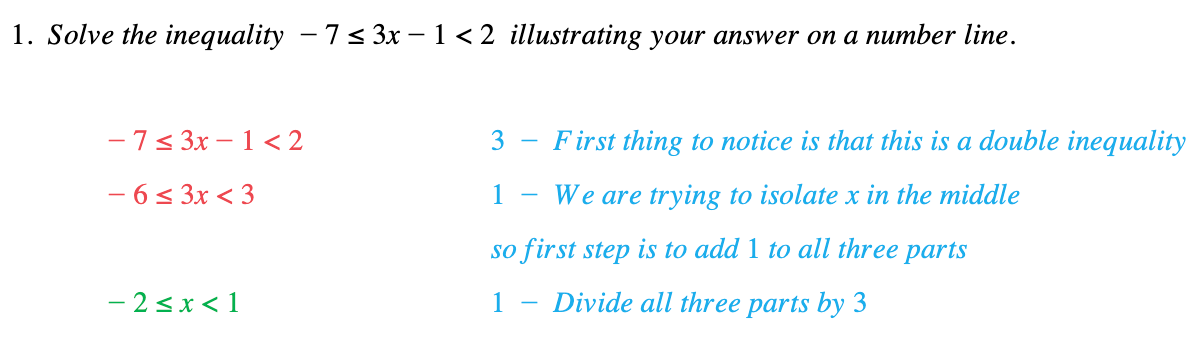# Edexcel IGCSE Maths 复习笔记 2.16.1 Solving Inequalities - Linear

Edexcel IGCSE Maths 复习笔记 2.16.1 Solving Inequalities - Linear

#### What is a linear inequality?

• An Inequality tells you that one expression is greater than (“>”) or less than (“<”) another
• “⩾” means “greater than or equal to”
• “⩽” means “less than or equal to”

• Linear Inequality just has an x (and/or a y) etc in it and no x2 or similar
• For example, 3x + 4 ⩾ 7 would be read “3x + 4 is greater than or equal to 7”

#### Solving linear inequalities

• Solving linear inequalities is just like Solving Linear Equations (so review these notes first)
• You also need to know how to use Number Lines and deal with “Double” Inequalities

1. Same rules as solving equations: GROF GROBLET FIND ANSWER!

But do NOT multiply (or divide) by negative numbers

2. When drawing NUMBER LINES:3. For “double” inequalities just do the same thing to all three parts

#### Worked Example